Data Science Desktop Survival Guide by Graham WilliamsDesktop Survival Project Home Preface Data Science Introducing R R Constructs R Tasks R Strings R Read, Write, and Create Data Template Data Exploration Data Wrangling Data Visualisation Statistics ML Template ML Scenarios ML Activities ML Applications ML Algorithms Cluster Analysis Decision Trees Computer Vision Graph Data Privacy Literate Data Science Coding with Style Resources Bibliography Index

## Naïve Bayes

Representation: Probabilities

Consider classifying input data by determining the probability of an event happening given the probability of another event that has already occured. Class and conditional probabilities are calculated to determine the likelihood of an event.

Naïve Bayes is a simple and effective machine learning classifier based on Bayes' Theorem: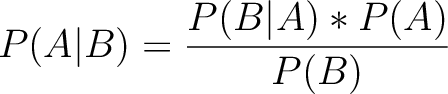The class probabilities are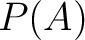(the probability of event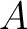) and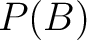. The conditional probabilities are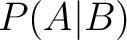(the probability ofhappening given that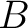occurs) and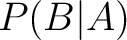.

A Naïve Bayes classifier uses these probabilities to classify independent features that have the same weight or importance from the input dataset to make predictions.

The idea is simple and can be applied to small datasets. It suffers from the zero frequency problem where a class with probability of 0 for a selected feature, and hence its conditional probability is then also 0 and so excluded from further consideration. Laplace smoothing assigns the class a small probability.

The concept also assumes independence of the features and when not met will perform poorly.